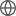Old Web
English
Boosting is a well-known method for improving the accuracy of weak learners in machine learning. However, its theoretical generalization guarantee is missing in literature. In this paper, we propose an efficient boosting method with theoretical generalization guarantees for binary classification. Three key ingredients of the proposed boosting method are: a) the \textit{fully-corrective greedy} (FCG) update in the boosting procedure, b) a differentiable \textit{squared hinge} (also called \textit{truncated quadratic}) function as the loss function, and c) an efficient alternating direction method of multipliers (ADMM) algorithm for the associated FCG optimization. The used squared hinge loss not only inherits the robustness of the well-known hinge loss for classification with outliers, but also brings some benefits for computational implementation and theoretical justification. Under some sparseness assumption, we derive a fast learning rate of the order ${\cal O}((m/\log m)^{-1/4})$ for the proposed boosting method, which can be further improved to ${\cal O}((m/\log m)^{-1/2})$ if certain additional noise assumption is imposed, where $m$ is the size of sample set. Both derived learning rates are the best ones among the existing generalization results of boosting-type methods for classification. Moreover, an efficient early stopping scheme is provided for the proposed method. A series of toy simulations and real data experiments are conducted to verify the developed theories and demonstrate the effectiveness of the proposed method.